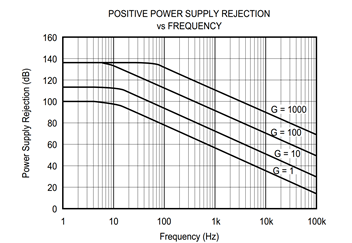Other Parts Discussed in Post: INA118

Electrical engineers are accustomed to dealing with rejection, and we absolutely love it. From common-mode rejection to power supply rejection, and even EMI rejection. The more rejection, the better!

However, in the case of instrumentation amplifiers, it’s easy to get confused when calculating the offset shift caused by a change in the power supply or common-mode voltage. The root cause of this confusion is plots like this one:Figure 1: A typical power supply rejection ratio curve for an instrumentation amplifier

In Figure 1, the power supply rejection ratio (PSRR) of the amplifier increases as the amplifier is configured for higher gains. It’s tempting to think that in high gains it would take a massive change in the power supply to cause any shift at the output! But remember that both common mode rejection ratio (CMRR) and PSRR are input-referred specifications:

PSRR and CMRR are defined as the change in the input offset voltage, ΔVOS(IN), divided by the change in the supply voltage, ΔVS, or common-mode voltage ΔVCM.

To understand how gain effects these specifications, consider that most instrumentation amplifiers are actually two amplifier stages in series – an input stage amplifier, shown in Figure 2 as G1, and an output stage amplifier, shown as G2. A change in the power supply or common mode voltage will cause a change in the offset of each of these amplification stages, which is shown as ΔVOS1 and ΔVOS2.

Figure 2: A conceptual diagram of most instrumentation amplifiers

The change in second offset ΔVOS2 is divided by the input stage gain G1 when it is referred to the input. Finally, because the polarities of the two shifts in offset are unknown, they may either add or subtract, leading to the format of equation 2:

You will see this format in instrumentation amplifier datasheets to specify the change in input offset due to different factors such as temperature, power supply, and common mode voltage:

Figure 3: Excerpt from the INA118 datasheet showing the change in input offset due to different factors.

By inserting equation 2 into equation 1, it now becomes apparent how gain affects the PSRR and CMRR of an instrumentation amplifier:

These specifications improve as gain increases, because the change in the second amplifier’s offset, ΔVOS2, is divided by the gain of the input stage.

So far, we’ve focused on changes in the input offset, but what happens at the output? After all, it’s usually the output of the amplifier that we really care about. Intuitively, we multiply the ΔVOS(IN) by the total gain of the amplifier to calculate ΔVOS(OUT).

Many instrumentation amplifiers have an output stage gain of 1, meaning the total gain of the amplifier is determined by the input stage gain. This allows us to simplify equation 4:

The CMRR and PSRR specifications of an instrumentation amplifier improve at higher gains, because the input stage is becoming the dominant source of error. But there is another effect that we haven’t discussed yet. The careful observer of figure 3 may have noticed that the output stage offset is worse than the input stage. Read my next blog to find out why!

Parents
• Excellent information, thank you.  I am looking at figure1 and read 2-3 times the equetions. Figure 1 is saying to me if PSRR of ina increases as the ina configured for higher gain. it is temting to think V(os1) and V(os2) must be same polarity.  Otherwise higher gain would get lower PSRR.

Comment
• Excellent information, thank you.  I am looking at figure1 and read 2-3 times the equetions. Figure 1 is saying to me if PSRR of ina increases as the ina configured for higher gain. it is temting to think V(os1) and V(os2) must be same polarity.  Otherwise higher gain would get lower PSRR.

Children
No Data